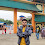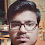# HackerRank Python If-Else problem solution

In this HackerRank Python If - Else problem-solution set, Given an integer, n, perform the following conditional actions:

• If n is odd, print Weird
• If n is even and in the inclusive range of 2 to 5, print Not Weird
• If n is even and in the inclusive range of 6 to 20, print Weird
• If n is even and greater than 20, print Not Weird

## Problem solution in Python 2 programming.

```#!/bin/python

import sys

N = int(raw_input().strip())

if (N%2):
print ("Weird")
elif (N>=2 and N<=5):
print ("Not Weird")
elif (N>=6 and N<=20):
print ("Weird")
else: print ("Not Weird")```

## Problem solution in Python 3 programming.

```#!/bin/python3

import math
import os
import random
import re
import sys

if __name__ == '__main__':
n = int(input().strip())

if n%2 != 0:
print("Weird")
else:
if n>=2 and n<=5:
print("Not Weird")
elif n>=6 and n<=20:
print("Weird")
else:
print("Not Weird")```

### Problem solution in pypy programming.

```# Enter your code here. Read input from STDIN. Print output to STDOUT
n = int(raw_input())

if n%2 == 1:
print('Weird')
elif n>=2 and n<=5:
print('Not Weird')
elif n>=6 and n<=20:
print('Weird')
elif n>20:
print('Not Weird')```

### Problem solution in pypy3 programming.

```# Enter your code here. Read input from STDIN. Print output to STDOUT
val = int(input())
output = "Weird"
if val%2 == 1:
pass
elif 2 <= val < 5:
output = "Not Weird"
elif 6 <= val <= 20:
pass
else:
output = "Not Weird"

print(output)```

1.bro give me explanation of this line
N = int(raw_input().strip())

2.the line means we are going to take an string as an integer input and then strip it means remove the white space around the string and then convert it into the integer variable.

3.if name=='durga':
print('hello durga,good evening')
print('how are you')
else:
print('hello durga,good evening')
print('Who are you')
why there is error comming in else

1.name = "durga"
if name =="durga":
print("hello durga,good evening")
print("How are you?")
else:
print("hello durga,good evening")
print("Who are you?")

4.if __name__ == '__main__':
n = int(input().strip())
if n % 3 == 0:
print ('Weird')
elif n%2 == 0 and 2 >= n <= 5:
print ( 'Not Weird')
elif n%2 == 0 and 6 >= n <= 20:
print ('Weird')
elif n%2 == 0 and n>20 :

print ('Not Weird')

when i take 24 it is not printing " not weird " , why?

5.There might be an error while submitting for 4,
for this in python3

#!/bin/python3

import math
import os
import random
import re
import sys

if __name__ == '__main__':
n = int(input().strip())

if n%2 != 0:
print("Weird")
elif n == 4:
print("Not Weird")
else:
if n>=2 and n<=5:
print("Not Weird")
if n>=6 and n<=20:
print("Weird")
else:
print("Not Weird")

6.nms=[]
choice='yes'

while choice=='yes':
n=input ('enter a name')
nms.append(n)
choice=input('do you want to continue?')
else:
print(nms)
Why is the error coming in this??